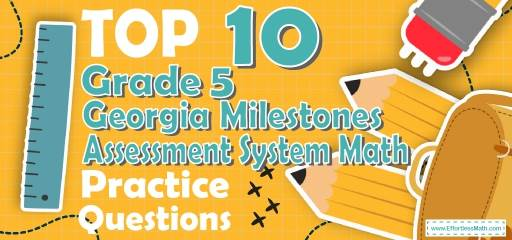# Top 10 5th Grade Georgia Milestones Assessment System Math Practice QuestionsThe best way to prepare for your 5th Grade Georgia Milestones Assessment System Math test is to work through as many 5th Grade Georgia Milestones Assessment System Math practice questions as possible. Here are the top 10 5th Grade Georgia Milestones Assessment System Math practice questions to help you review the most important 5th Grade Georgia Milestones Assessment System Math concepts. These 5th Grade Georgia Milestones Assessment System Math practice questions are designed to cover mathematics concepts and topics that are found on the actual test. The questions have been fully updated to reflect the latest 2022 5th Grade Georgia Milestones Assessment System guidelines. Answers and full explanations are provided at the end of the post.

Start your 5th Grade Georgia Milestones Assessment System Math test prep journey right now with these sample 5th Grade Georgia Milestones Assessment System Math questions.

## 5th Grade Georgia Milestones Assessment System Math Practice Questions

1- In a bag, there are 65 cards. Of these cards, 13 cards are white. What fraction of the cards are white?

A. $$\frac{1}{5}$$

B. $$\frac{2}{7}$$

C. $$\frac{13}{52}$$

D. $$\frac{1}{4}$$

2- Which expression is equal to $$\frac{3}{11}$$ ?

A. $$3 – 11$$

B. $$3 ÷ 11$$

C. $$3 × 11$$

D. $$\frac{11}{3}$$

3- If $$A = 20$$, then which of the following equations are correct?

A. $$A + 20 = 40$$

B. $$A ÷ 20 = 40$$

C. $$20 × A = 40$$

D. $$A – 20 = 40$$

4- How long does a 425 miles trip take moving at 50 miles per hour (mph)?

A. 4 hours

B. 6 hours and 24 minutes

C. 8 hours and 24 minutes

D. 8 hours and 30 minutes

5- 50 is What percent of 40?

A. $$20 \%$$

B. $$25 \%$$

C. $$125 \%$$

D. $$150 \%$$

6- The perimeter of the trapezoid below is 52. What is its area? _________

7- The area of a circle is $$64π$$. What is the circumference of the circle?

A. $$8 π$$

B. $$16 π$$

C. $$32 π$$

D. $$64 π$$

8- A rope weighs 800 grams per meter of length. What is the weight in kilograms of 12.2 meters of this rope? (1 kilograms = 1000 grams)

A. 0.0976

B. 0.976

C. 9.76

D. 9760

9- Solve.
$$\frac{1}{2} + \frac{4}{5} – \frac{3}{10} = ?$$

A. $$\frac{9}{10}$$

B. $$\frac{2}{10}$$

C. 1

D. 2

10- How many $$\frac{1}{4}$$ cup servings are in a package of cheese that contains $$6 \frac{1}{2}$$ cups altogether?_________

## Best 5th Grade Georgia Milestones Assessment System Math Prep Resource for 2022

1- A
There are 65 cards in the bag and 13 of them are white. Then, 13 out of 65 cards are white. You can write this as: $$\frac{13}{65}$$.
To simplify this fraction, divide both numerator and denominator by 13. Then:
$$\frac{13}{65}=\frac{1}{5}$$

2- B
$$\frac{3}{11}$$ means 3 is divided by 11. The fraction line simply means division or $$÷$$. Therefore, we can write $$\frac{3}{11}$$ as $$3 ÷ 11$$.

3- A
Plugin 20 for A in the equations. Only option A works.
$$A+20=40$$
$$20+20=40$$

4- D
50 miles : 1 hour
425 miles : $$425 ÷ 50 = 8.5$$ hours

5- C
Use percent formula:
$$part =\frac{percent}{100}× whole$$
$$50=\frac{percent}{100}× 40⇒ 50 = \frac{percent ×40}{100}⇒ 50 = \frac{percent ×4}{10}$$
multiply both sides by 10.
500 = percent $$×4$$, divide both sides by 4.
125 = percent

6- 104
First, find the missing side of the trapezoid. The perimeter of the trapezoid below is 52. Therefore, the missing side of the trapezoid (its height) is: $$52-12-18-14=52-44=8$$
Area of a trapezoid: $$A = \frac{1}{2} h (b_{1} + b_{2}) = \frac{1}{2} (8) (12 + 14) = 104$$

7- B
Use area and circumference of a circle formula.
Area of a circle = $$πr^2 ⇒ 64π = πr^2 ⇒ r= 8$$
Circumference of a circle = $$2πr ⇒ C = 2×8×π ⇒ C =16π$$

8- C
1 meter of the rope = 800 grams 12.2 meter of the rope = $$12.2 × 800 =9,760$$grams = 9.76 kilograms

9- C
$$\frac{1}{2} + \frac{4}{5} – \frac{3}{10} = \frac{(5×1)+(2×4)-(1×3)}{10} =\frac{10}{10}=1$$

10- 26
To solve this problem, divide $$6\frac{1}{2}$$ by $$\frac{1}{4}$$.
$$6\frac{1}{2}÷\frac{1}{4}=\frac{13}{2}÷\frac{1}{4}=\frac{13}{2}×\frac{4}{1}=26$$

Looking for the best resource to help you succeed on the 5th Grade Georgia Milestones Assessment System Math test?

## The Best Books to Ace the 5th Grade Georgia Milestones Assessment System Math Test

### What people say about "Top 10 5th Grade Georgia Milestones Assessment System Math Practice Questions - Effortless Math: We Help Students Learn to LOVE Mathematics"?

No one replied yet.

X
27% OFF

Limited time only!

Save Over 27%

SAVE $5 It was$18.99 now it is \$13.99